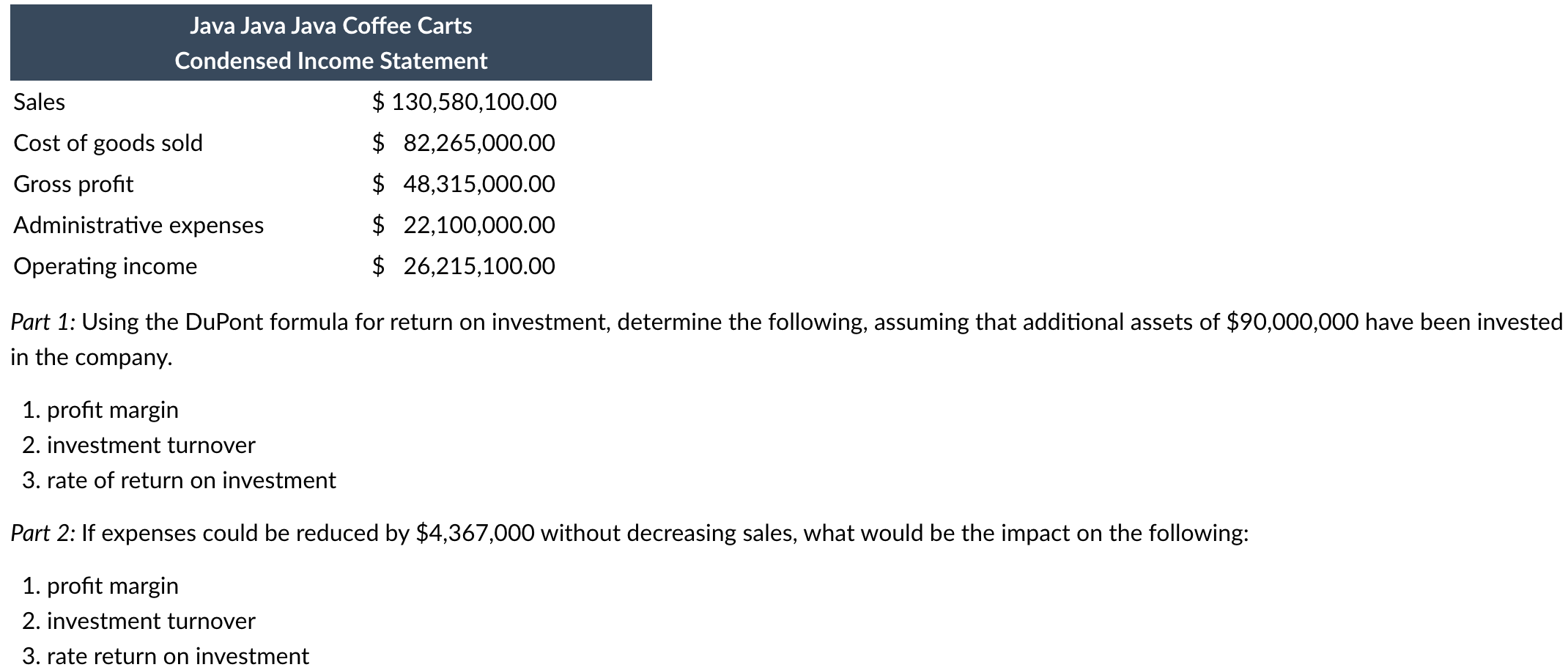Home / Expert Answers / Accounting / part-1-using-the-dupont-formula-for-return-on-investment-determine-the-following-assuming-that-a-pa270

# (Solved): Part 1: Using the DuPont formula for return on investment, determine the following, assuming that a ...Part 1: Using the DuPont formula for return on investment, determine the following, assuming that additional assets of have been invested in the company. 1. profit margin 2. investment turnover 3. rate of return on investment Part 2: If expenses could be reduced by without decreasing sales, what would be the impact on the following: 1. profit margin 2. investment turnover 3. rate return on investment

We have an Answer from Expert